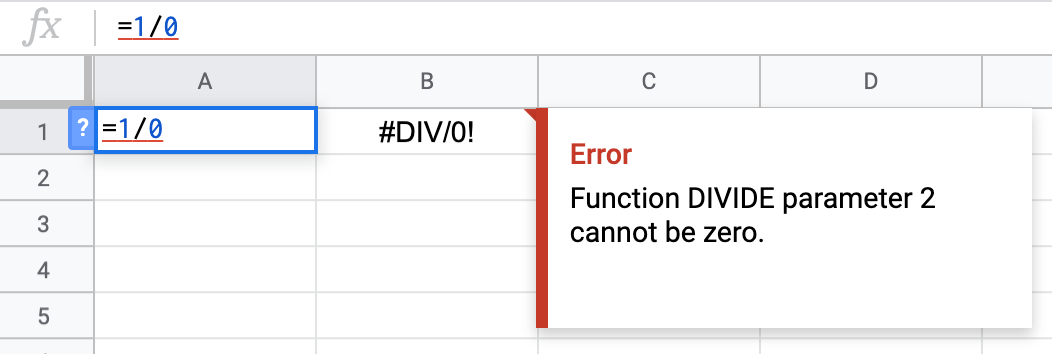# Errors¶

If Sheets cannot interpret your formula or your formula cannot be computed, you will see an error. An example of this is below. Cell A1 is the formula, B1 is the result. Note the red line under A1 indicating Sheets will throw an error here. The box entitled `Error` tells you why Sheets could not compute the formula.There are several types of errors, and this section does not cover them in depth. Throughout this course, you will undoubtedly come across such errors, and that is absolutely normal. Not encountering errors would indeed be suspicious! In general, there is no foolproof way to debug these errors, but some useful tips are below.

1. Look at the error message. In the above example, “Function DIVIDE parameter 2 cannot be zero” is telling you that the second value (the value after the division symbol “/”) cannot be zero, since dividing by zero is infinite or undefined mathematically.

2. If you are using a function, know the inputs. This table, written by Google, lists all functions and how to set the inputs. The table is ordered by “type”, so you can find common mathematical functions with the type “Math”.

3. Try Googling your error. If you are facing an error, chances are several people have seen it before. Try searching for the error message or the broken formula online. Sites like Google Support and StackOverflow have helped millions.

4. Practice makes perfect. No matter what you do, you will see errors. Debugging errors is a big part of coding, but the more you practice, the fewer errors you will see.

You have attempted of activities on this page